Home
Hostname: page-component-cf9d5c678-h2mp8 Total loading time: 0.301 Render date: 2021-07-28T08:50:24.693Z Has data issue: true Feature Flags: { "shouldUseShareProductTool": true, "shouldUseHypothesis": true, "isUnsiloEnabled": true, "metricsAbstractViews": false, "figures": true, "newCiteModal": false, "newCitedByModal": true, "newEcommerce": true, "newUsageEvents": true }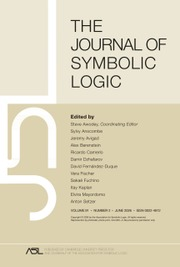The Journal of Symbolic Logic

# THE RELATION BETWEEN TWO DIMINISHED CHOICE PRINCIPLES

Part of: Set theory

Published online by Cambridge University Press:  15 February 2021

Corresponding

## Abstract

For every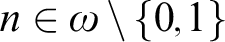$n\in \omega \setminus \{0,1\}$ we introduce the following weak choice principle: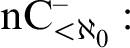$\operatorname {nC}_{<\aleph _0}^-:$ For every infinite family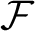$\mathcal {F}$ of finite sets of size at least n there is an infinite subfamily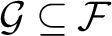$\mathcal {G}\subseteq \mathcal {F}$ with a selection function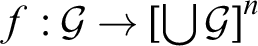$f:\mathcal {G}\to \left [\bigcup \mathcal {G}\right ]^n$ such that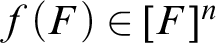$f(F)\in [F]^n$ for all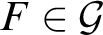$F\in \mathcal {G}$ .

Moreover, we consider the following choice principle: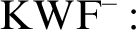$\operatorname {KWF}^-:$ For every infinite family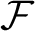$\mathcal {F}$ of finite sets of size at least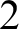$2$ there is an infinite subfamily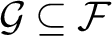$\mathcal {G}\subseteq \mathcal {F}$ with a Kinna–Wagner selection function. That is, there is a function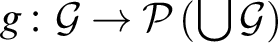$g\colon \mathcal {G}\to \mathcal {P}\left (\bigcup \mathcal {G}\right )$ with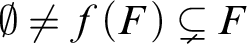$\emptyset \not =f(F)\subsetneq F$ for every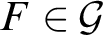$F\in \mathcal {G}$ .

We will discuss the relations between these two choice principles and their relations to other well-known weak choice principles. Moreover, we will discuss what happens when we replace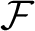$\mathcal {F}$ by a linearly ordered or a well-ordered family.

## MSC classification

Type
Article
Information
The Journal of Symbolic Logic , March 2021 , pp. 415 - 432
© The Association for Symbolic Logic 2021

## References

De La Cruz, O. and Di Prisco, C. A., Weak forms of the axiom of choice and partitions of infinite sets, Set Theory (Di Prisco, C. A., Larson, J. A., Bagaria, J., and Mathias, A. R. D., editors), Springer, Dordrecht, 1998, pp. 4770.CrossRefGoogle Scholar
De La Cruz, O. and Di Prisco, C. A., Weak choice principles. Proceedings of the American Mathematical Society, vol. 126 (1998), no 3, pp. 867876.CrossRefGoogle Scholar
Halbeisen, L., Combinatorial Set Theory—With a Gentle Introduction to Forcing, second ed., Springer Monographs in Mathematics, Springer International Publishing, London, 2017.Google Scholar
Halbeisen, L., Plati, R., and Schumacher, S., A new weak choice principle, preprint, 2021, arXiv:2101.07840.Google Scholar
Halbeisen, L. and Schumacher, S., Some implications of Ramsey choice for n-element sets, preprint, 2021, arXiv:2101.06924.Google Scholar
Halbeisen, L. and Tachtsis, E., On Ramsey choice and partial choice for infinite families of n-element sets. Archive for Mathematical Logic, vol. 59 (2020), pp. 583606.CrossRefGoogle Scholar
Howard, P., Rubin, A. L., and Rubin, J. E., Kinna–Wagner selection principles, axiom of choice and multiple choice. Monatsheft für Mathematik, vol. 123 (1997), pp. 309319.CrossRefGoogle Scholar
Howard, P. and Rubin, J. E., Consequences of the Axiom of Choice, Mathematical Surveys and Monographs, vol. 59, American Mathematical Society, Providence, RI, 1998.CrossRefGoogle Scholar
Keremedis, K. and Wajch, E., Denumerable cellular families in Hausdorff spaces and towers of Boolean algebras in ZF, preprint, 2020, arXiv:2001.00619.Google Scholar
Lévy, A., Axioms of multiple choice. Fundamenta Mathematicae, vol. 50 (1962), no. 5, pp. 475485.CrossRefGoogle Scholar
Montenegro, C. H., Weak versions of the axiom of choice for families of finite sets, Models, Algebras, and Proofs (Caicedo, X. and Montenegro, C., editors), Lecture Notes in Pure and Applied Mathematics, vol. 203, Dekker, New York, 1999, pp. 5760.Google Scholar
Pincus, D., Zermelo–Fraenkel consistency results by Fraenkel–Mostowski methods, this Journal, vol. 37 (1972), pp. 721743.Google ScholarYou have Access

# Send article to Kindle

Note you can select to send to either the @free.kindle.com or @kindle.com variations. ‘@free.kindle.com’ emails are free but can only be sent to your device when it is connected to wi-fi. ‘@kindle.com’ emails can be delivered even when you are not connected to wi-fi, but note that service fees apply.

Find out more about the Kindle Personal Document Service.

THE RELATION BETWEEN TWO DIMINISHED CHOICE PRINCIPLES
Available formats
×

# Send article to Dropbox

To send this article to your Dropbox account, please select one or more formats and confirm that you agree to abide by our usage policies. If this is the first time you use this feature, you will be asked to authorise Cambridge Core to connect with your <service> account. Find out more about sending content to Dropbox.

THE RELATION BETWEEN TWO DIMINISHED CHOICE PRINCIPLES
Available formats
×

# Send article to Google Drive

To send this article to your Google Drive account, please select one or more formats and confirm that you agree to abide by our usage policies. If this is the first time you use this feature, you will be asked to authorise Cambridge Core to connect with your <service> account. Find out more about sending content to Google Drive.

THE RELATION BETWEEN TWO DIMINISHED CHOICE PRINCIPLES
Available formats
×
×